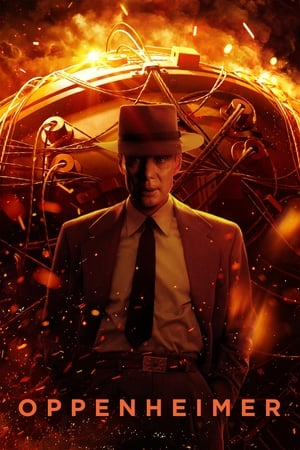Genre
Drama
Year
2023
Runtime
3h01

# Oppenheimerself.__wrap_b=(g,_,x)=>{let w=(x=x||document.querySelector(`[data-br="\${g}"]`)).parentElement,O=j=>x.style.maxWidth=j+"px";x.style.maxWidth="";let M=w.clientWidth,L=w.clientHeight,B=M/2,Q=M,oe;if(M){for(;B+1<Q;)O(oe=~~((B+Q)/2)),w.clientHeight===L?Q=oe:B=oe;O(Q*_+M*(1-_))}x.__wrap_o||(x.__wrap_o=new ResizeObserver(()=>{self.__wrap_b(0,+x.dataset.brr,x)})).observe(w)};self.__wrap_b(":R6llddda:",1)

## Synopsys

The story of J. Robert Oppenheimer's role in the development of the atomic bomb during World War II.

## Cast

•### Cillian Murphy

J. Robert Oppenheimer

•### Emily Blunt

Kitty Oppenheimer

•### Matt Damon

Leslie Groves

•### Robert Downey Jr.

Lewis Strauss

•### Florence Pugh

Jean Tatlock

•### Josh Hartnett

Ernest Lawrence